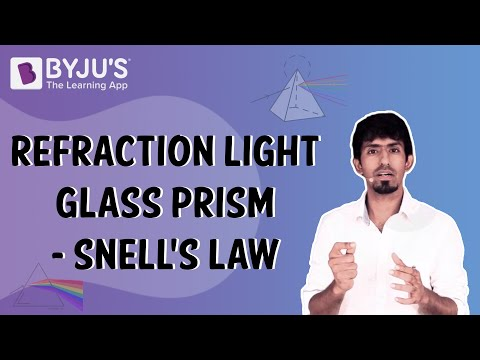# Class 12 Physics Chapter 9 Ray Optics and Optical Instruments MCQs

Access the latest Class 12 Physics MCQs for Chapter 9 Ray Optics and Optical Instruments, along with their answers. The MCQs with answers for Class 12 Physics are created as per the latest exam pattern. These questions will help students improve their critical thinking abilities and help them score good marks in the board exams.

Download Chapter 9 Ray Optics and Optical Instruments MCQs PDF by clicking on the button below.

## Class 12 Ray Optics and Optical Instruments MCQs

Check the multiple-choice questions for the 12th Class Physics Optical Instruments Chapter. Each MCQ will have four options here, out of which only one is correct. Students have to pick the correct option and check the answer provided here.

1. Which of the following lights deviates the most when it passes through a prism?

1. Red Light
2. Violet Light
3. Neither (a) nor (b)
4. Both (a) and (b)

Explanation: Violet Light deviates the most when it passes through a prism.

2. Which of the following phenomena of light results in a mirage?

1. Refraction of light
2. Reflection of light
3. Total internal reflection
4. (d) Diffraction of light

Explanation: Total Internal Reflection results in a mirage.

3. For which of the following is the field of view maximum?

1. Concave mirror
2. Convex mirror
3. Plane mirror
4. Cylindrical mirror

Explanation: For convex mirrors, the field of view is maximum.

4. What happens when the light is refracted into a medium?

1. Both frequency and wavelength of the light increases
2. The wavelength increases but the frequency remain unchanged
3. Both wavelength and frequency decreases
4. The wavelength decreases but the frequency remain constant

Answer: (b) The wavelength increases but the frequency remains unchanged

Explanation: When the light is refracted into a medium its wavelength increases but the frequency remains unchanged.

5. If a glass prism is dipped in water, what happens to its dispersive power?

1. Increases
2. Decreases
3. Does not change
4. No effect

Explanation: If a glass prism is dipped in water, its dispersive power decreases.

6. What should be increased to increase the angular magnification of a simple microscope?

1. The power of the lens
2. The focal length of the lens
3. Lens Aperture
4. Object Size

Answer: (a) The power of the lens

Explanation: The power of the lens should be increased to increase the angular magnification of a simple microscope.

7. Which of the following phenomenon is used in optical fibre?

1. Refraction
2. Diffraction
3. Scattering
4. Total Internal Reflection

Explanation: Total Internal Reflection is used in optical fibre.

8. Which of the following statements is true of total internal reflection?

1. Light travels from rarer medium to denser medium
2. Light travels from denser medium to rarer medium
3. Light travels in water only
4. Light travels in the air only

Answer: (b) Light travels from denser medium to rarer medium

Explanation: In total internal reflection, the light travels from a denser medium to a rarer medium.

9. A convex lens is dipped in a liquid whose refractive index is equal to the refractive index of the lens. Then what is its focal length?

1. Focal Length will become zero
2. Focal Length will become infinite
3. Focal length will reduce, but not become zero
4. Remains unchanged

Answer: (b) Focal Length will become infinite

Explanation: The focal length of the lens becomes infinite.

10. For a telescope, the larger the diameter of the objective lens

1. Greater the resolving power
2. Greater the magnifying power
3. Smaller the resolving power
4. Smaller the magnifying power

Answer: (b) Greater the resolving power

Explanation: For a telescope, the larger the diameter of the objective lens, the greater the resolving power.

## Watch the video to visualise the refraction of light through a glass prism.Stay tuned to BYJU’S and Fall in Love with Learning!